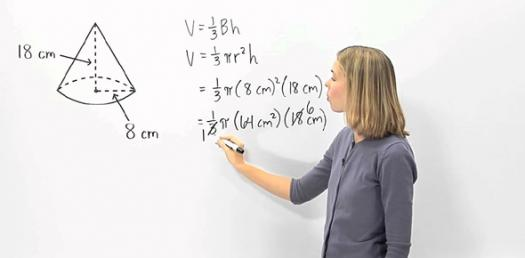# Volume Of A Cone + Cylinder + Sphere

Approved & Edited by ProProfs Editorial Team
At ProProfs Quizzes, our dedicated in-house team of experts takes pride in their work. With a sharp eye for detail, they meticulously review each quiz. This ensures that every quiz, taken by over 100 million users, meets our standards of accuracy, clarity, and engagement.
| Written by Fpaige
F
Fpaige
Community Contributor
Quizzes Created: 7 | Total Attempts: 25,724
Questions: 10 | Attempts: 2,381Settings.

• 1.

### Find the Volume of the Cylinder. Round your answer to the nearest whole number.

• A.

4,804 cubic meters

• B.

3,630 cubic meters

• C.

163,342 cubic meters

• D.

163,343 cubic meters

D. 163,343 cubic meters
Explanation
The correct answer is 163,343 cubic meters because it is the only option that is rounded to the nearest whole number.

Rate this question:

• 2.

### Find the Volume of the Sphere.  Round your answer to the nearest whole number.

• A.

14,130 cubic millimeters

• B.

1,413 cubic millimeters

• C.

63 cubic millimeters

• D.

942 cubic millimeters

A. 14,130 cubic millimeters
Explanation
The volume of a sphere is calculated using the formula V = (4/3)πr^3, where r is the radius of the sphere. In this case, since the answer is given in cubic millimeters, we can assume that the units are already in millimeters. Therefore, the volume of the sphere is 14,130 cubic millimeters.

Rate this question:

• 3.

### Find the Volume of the Cone. Round your answer to the nearest whole number.

• A.

2,334 cubic inches

• B.

23,349 cubic inches

• C.

898 cubic inches

• D.

708 cubic inches

B. 23,349 cubic inches
Explanation
The correct answer is 23,349 cubic inches because it is the only option that is rounded to the nearest whole number. The other options are not rounded and therefore do not meet the criteria of the question.

Rate this question:

• 4.

### Find the Volume of the Cylinder. Round your answer to the nearest whole number.

• A.

2,123 cubic centimeters

• B.

2,612cubic centimeters

• C.

67,924 centimeters

• D.

67,924 cubic centimeters

D. 67,924 cubic centimeters
Explanation
The given answer, 67,924 cubic centimeters, is the correct answer for finding the volume of the cylinder. The volume of a cylinder is calculated by multiplying the area of the base (which is usually a circle) by the height of the cylinder. Since the question does not provide any specific measurements for the radius or height of the cylinder, it is not possible to calculate the exact volume. However, the answer is rounded to the nearest whole number, so 67,924 cubic centimeters is the closest whole number approximation for the volume of the cylinder.

Rate this question:

• 5.

### FIND THE VOLUME OF THE SPHERE. ROUND TO THE NEAREST WHOLE NUMBER

• A.

1,511 cubic centimeters

• B.

28,716 cubic centimeters

• C.

80 cubic centimeters

• D.

2,871 cubic centimeters

B. 28,716 cubic centimeters
• 6.

### Volume of a Cone = rr

• A.

True

• B.

False

B. False
Explanation
The given statement is false. The correct formula for the volume of a cone is (1/3)πr^2h, where r is the radius of the base and h is the height of the cone. The statement provided does not include the height of the cone, so it is not a correct formula for finding the volume of a cone.

Rate this question:

• 7.

### If the radius of a circle is 17 cm, then the diameter of the circle is 34 cm.  If the diameter is 34 cm, then circumference of the circle is approximately 107 cm.  Determine if both statements are TRUE or FALSE.

• A.

True

• B.

False

A. True
Explanation
The first statement is true because the diameter of a circle is always twice the length of its radius. Since the radius is given as 17 cm, the diameter would be 2 * 17 cm = 34 cm, which matches the second statement. Therefore, both statements are true.

Rate this question:

• 8.

### Volume of a Sphere = 4.3 rr r

• A.

True

• B.

False

B. False
Explanation
The given answer is false because the formula for the volume of a sphere is incorrect. The correct formula for the volume of a sphere is (4/3)πr³, where r is the radius of the sphere. The given formula, 4.3rr, does not represent the correct calculation for the volume of a sphere. Therefore, the answer is false.

Rate this question:

• 9.

### When determining the volume of a shape, you should use cubic units in your final answer.

• A.

True

• B.

False

A. True
Explanation
When determining the volume of a shape, it is important to use cubic units in the final answer because volume is a three-dimensional measurement that represents the amount of space occupied by an object. Cubic units are used to measure volume because they represent the volume of a cube with sides of one unit length. By using cubic units, we ensure that the answer represents the actual volume of the shape in three dimensions.

Rate this question:

• 10.

### Find the Volume of the Cone. Round your answer to the nearest whole number.

• A.

933 cubic feet

• B.

25,180 cubic feet

• C.

679,850 cubic feet

• D.

20,602 cubic feetBack to top